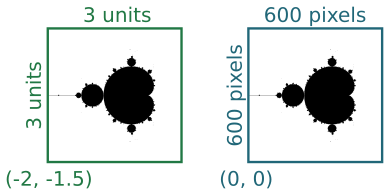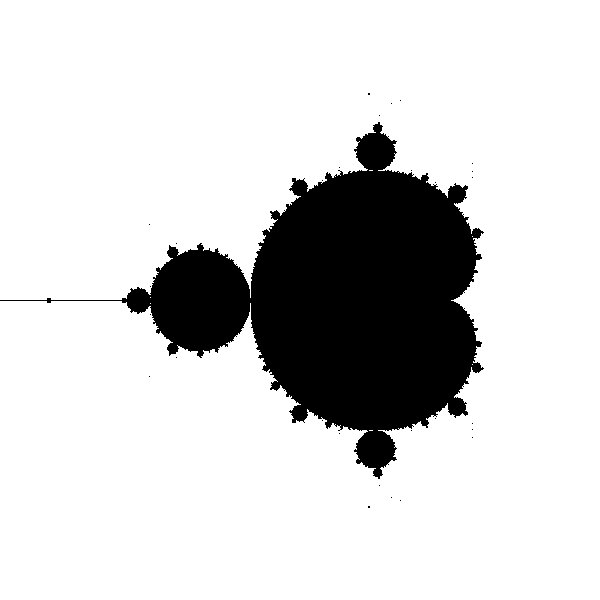# Black and white Mandelbrot fractal in generativepy

Martin McBride
2021-12-14

The Mandelbrot set is a famous fractal that can be implemented fairly easily with generativepy.

The Mandelbrot set is an escape-time fractal. This article will explain how escape-time fractals work, using the Mandelbrot as an example.

## Escape-time fractals

The Mandelbrot set is based on an equation using complex numbers:

znext = z*z + c


Where z is a complex variable, and c is a complex number constant. However, if you are not familiar with complex numbers we can rewrite this equation as a pair of equations in x and y:

xnext = x*x - y*y + c1
ynext = 2*x*y + c2


Where c1 and c2 are two constant values.

Here are those equations implemented in a Python loop:

def calc(c1, c2):
x = y = 0
for i in range(10):
x, y = x*x - y*y + c1, 2*x*y + c2
print(x, y)


We are interested in what happens as we call this function iteratively, with various values of c1 and c2. Now if we try calling this function with c1 = 0.2 and c2 = 0.1, the sequence of values looks like this:

0.2 0.1
0.23 0.14
0.2333 0.16440000000000002
0.22740153000000002 0.17670904
0.22048537102861931 0.18036781212166242
0.21608125118807261 0.17953692795453008
0.21445759861565283 0.17758912805375543
0.21445416320109933 0.1761706758853121
0.21495448107239606 0.1755610697551134
0.2153837397195433 0.17547527729145027


After a few loops, the values seem to stabilise - they converge. We could run the loop many more times, the values would stay almost the same forever.

If we try different values such as c1 = 0.5 and c2 = 1.0, we get something very different:

0.5 0.01
0.7499 0.02
1.06195001 0.039996000000000004
1.6261381437230003 0.09494750519992001
3.135310233727196 0.31879551971385567
10.228539678324857 2.0090457108504634
101.08675928277933 41.10920753800466
8529.065957891833 8311.20313340019
3668869.0894282013 141773599.43841496
-2.0086292897328776e+16 1040297553353152.1


The numbers get very big, very quickly - they diverge. If we ran the loop more times, the numbers would just get bigger and bigger.

## The Mandelbrot set

If we try this experiment with lots of different values for c1 and c2, we will find that some values converge and some diverge.

The set of all converging points is called the Mandelbrot set.

We could make an image, where each pair of values c1, c2 is represented by the pixel at (c1, c2). We could mark that pixel black if the values converge, white if they don't. To create a complete image, we would perform that calculation for every pixel in the image, using its coordinates to set the values of (c1, c2).

Since the equations are so simple, you might expect the shape created by the black pixels to also be simple - maybe a circle or something like that. But in fact, the shape is incredibly intricate, the edge of the shape is so complex that you can zoom in on it forever and never run out of finer and finer detail.

To plot this shape, we will first tidy up our calc function:

MAX_COUNT = 256

def calc(c1, c2):
x = y = 0
for i in range(MAX_COUNT):
x, y = x*x - y*y + c1, 2*x*y + c2
if x*x + y*y > 4:
return i+1
return 0


The main job of this function is to decide whether a particular point (c1, c2) will converge or diverge. We base this on two criteria:

1. If the value of x*x + y*y becomes greater than 4, it can be proven that the value will definitely diverge, so it isn't necessary to continue the loop.
2. If the value hasn't exceeded 4 after 256 iterations, then we will assume it is not going to diverge.

In the first case, we return the current loop count + 1 (which will be a value between 0 and 256). In the second case, we return 0.

## Plotting the image

We are going to make a 2-dimensional plot of all the points in the Mandelbrot set. For each pixel at position (x, y):

• Setting c1 = x and c2 = y, check if the sequence diverges.
• Make the point (x, y) black if the converges, and white if it diverges.

We simply repeat that procedure for every pixel in the image and plot the result.

It turns out that the interesting part of the Mandelbrot set is in a region that is 3 units square, with its bottom corner at (-2, -1.5). In other words, x values between -2 and +1, and y values between -1.5 and +1.5. We will create an image 600 pixels square that represents this region:The two squares represent the same region, measured in different coordinate spaces We call the first set (the 3 by 3 square) our userspace, and the second set (the 600 by 600 square) our pixel space.

We will use a Scaler object to convert between user space and pixel space. We create the scaler using the parameters above, then we can simply call:

x, y = scaler.device_to_user(px, py)


to convert pixel coordinates to user coordinates.

## The drawing code

Here is the full drawing code:

from generativepy.bitmap import Scaler
from generativepy.nparray import make_nparray

MAX_COUNT = 256

def calc(c1, c2):
x = y = 0
for i in range(MAX_COUNT):
x, y = x*x - y*y + c1, 2*x*y + c2
if x*x + y*y > 4:
return i+1
return 0

def paint(image, pixel_width, pixel_height, frame_no, frame_count):
scaler = Scaler(pixel_width, pixel_height, width=3, startx=-2, starty=-1.5)

for px in range(pixel_width):
for py in range(pixel_height):
x, y = scaler.device_to_user(px, py)
count = calc(x, y)
if count==0:
image[py, px] = 0

make_nparray('mandelbrot-bw.png', paint, 600, 600, channels=1)


This code is available on github in blog/fractals/mandelbrot-bw.py.

First looking at the top level, we are using the make_nparray function to create a bitmap image. This function accepts a paint function that does the actual drawing. The paint function is called with a NumPy array as its first parameter. Since we have defines the image to have one channel (ie a greyscale image) we only need to set one byte for each pixel

Within the paint function, we create a Scaler object using the pixel width and height, and the userspace width and offsets as mentioned above.

Now we have the main loop, which loops over every pixel in the output image. On each pass through the loop, px and py indicate the pixel coordinates of the current pixel. Here is the body of the main loop:

x, y = scaler.device_to_user(px, py)
count = calc(x, y)
if count==0:
image[py, px] = 0


First, we use the scaler to convert the pixel coordinates into user coordinates. px which has a pixel range 0 to 599 is converted to a user range of -2.0 to +1.0. py which also has a pixel range of 0 to 599 is converted to a user range of 1.5 to -1.5.

We call calc to find out if the point is within the Mandelbrot set. Recall that the value is 0 for points that are in the set, and greater than zero for points outside. The array is initialised to white, so we set the colour to black if the count is 0, or leave it white if not.

Here is the image created:## MAX_COUNT

Most of the points that are outside the Mandelbrot set will diverge very quickly. It will only take a few iterations for the value to reach the point where x*x + y*y is greater than 4, so we know for certain it has diverged.

Generally, the closer a point is to the boundary of the set, the longer it will take to diverge. Points that are very close to the border might take a large number of iterations to fully escape. There is no real limit to how many iterations it might take.

To avoid potentially looping for a very long time, we set a maximum number of iterations. If the point hasn't diverged after that number of iterations, we assume it is part of the set.

A MAX_COUNT of 256 is perfectly good for the whole fractal in a 600-pixel image. If you decided to zoom in to a small part of the fractal, you might see some blockiness around the edge of the fractal. If that happens you can increase MAX_COUNT.